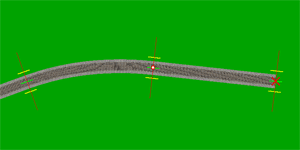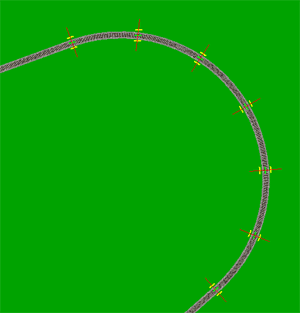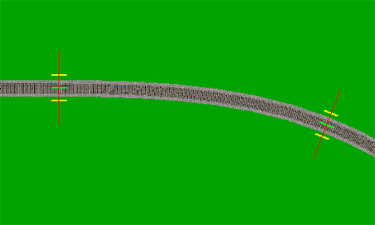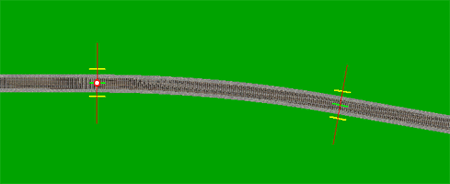# Rail3D*

 User Name: Password: Remember me next time.

# Laying Nice Curves

If you lay track with the Digitiser Tool, you often get unrealistically wiggly lines. How can you avoid this and get that professional look?

## 1 Prototype track-laying fundamentals

• Track sections are either straight lines or arcs of predetermined radius
• There is a strict minimum radius for a given line and type of rolling stock
• Sudden changes of curve radius are not allowed — a “transition curve” is used to reduce lateral jerk when trains go from straight to curved track and vice-versa
• “S”-curves are not allowed — there has to be at least a short straight between two curves of opposite hand. Normally this should be at least one vehicle long, to reduce the risk of buffer-locking.

## 2 Track-laying in Rail3D

Rail3D uses so-called Bezier curves instead of fixed geometry track. This produces the smoothest possible curve between any two nodes. It doesn’t worry about minimum radii, and it rarely produces a perfect straight-line unless you tell it to. If you’re laying track with the digitiser you will never get straight lines. Your track will be a succession of reverse curves, which, as we have seen, are an anathema in real life.

Why does Rail3D work this way? Would it not be more obvious to use a fixed geometry system like Microsoft Train simulator?

If you’ve ever tried laying track with msts, you will know that it is a very tedious and difficult business to get it right. When you do get it right, the results can be very good, but more often than not you will end up building the track which fits in with the pieces available rather than the track you want to build. The Bezier system used in Rail3D allows you to lay track very much more quickly, and to get something which is closer to the real geographical situation, but it does have certain limitations, as we have seen.

## 3 Getting better results with Rail3D

If you’re laying track directly on the screen, then the best advice is to take advantage of all the tracklaying aids which Rail3D provides. In particular, lay the straight sections first, then link them up with curves. If you’re laying more than one track, get the first track right first, then lay the second one parallel to it.

If you’re laying track with the digitiser, try to use as few nodes as possible. Place nodes at the beginning and end of straight sections. When you’ve laid a section of track with the digitiser, look at it carefully on the screen. If a section of track is meant to be straight, either straighten it using the Straighten between Markers tool, or, if it is relatively short, just lay new straight section on top of it and delete the original.

### 3.1 Circles, not ellipses

Especially in situations where you need relatively sharp curves (e.g. trams, narrow-gauge lines), it looks very bad if a curved section has a noticeably different radius at its two ends.Exaggerated example!

To correct this sort of thing, you first need to create “guide lines” by extending the tangents to the two ends of the curve until they cross. This is easy to do using the “snap straight” function in Rail3D.In a symmetrical, circular arc, these tangents should be equal in length. Thus, to correct the problem, you need to insert a new node C, such that the distance oc is roughy equal to ao.Lay a curve between A and C, and delete the old curve and guide lines.### 3.2 Dealing with “S”-curves

Generally, you will find that the digitiser has created quite a few reverse curves. These are usually created as single links.The best way to deal with these is to start by laying a new straight link over the top of the reverse curve.The idea is to build nice, smooth curve sections to transition between the straights. As a guide, extend the straights on either side to cross the “diagonal” (I’ve deleted the original “S”-link to make it easier to see what I’m doing.)Insert nodes in the diagonal roughly the same distance from the intersection as the nodes on the original straights.Join the nodes with curves, and delete the various “construction lines”The result looks much better!### 3.3 Laying long corners

#### Building a corner in stages

As we have seen, the best way to lay a corner is to do the straight parts first, and then try to make the curved section as circular as possible, not elliptical. But if you have a really long corner, it’s harder to guess distances correctly and you may find it easier to do it in stages. The only problem with laying track in stages is that you have to keep the radius of the corner constant, otherwise a train traversing the curve might find that the curve tightens suddenly.

We’re going to build this curve using straight sections of track positioned strategically so that we can join them all up to make a nice curve. To do this, we’ll need to make use of the length and angle information that Rail3D tells us when we lay track.

To build one big curve in stages, we’re going to do several little “units” of curve. Each of these units will have two straight “construction” pieces of track (which will be deleted eventually) and one curve track that makes up part of the overall curve. For convenience, I’ll refer to the two construction tracks in each unit as the A and B sections respectively.

To start, we lay the A section of the first unit. I’ve chosen it to be 40.0m long, and we’ll have to remember this length (let’s call it the section length). Note also that we’re laying at 70°.We’ll now lay another straight section of track over the first - this one is the B section - to help us build the curve with an even radius. Note how I have used the ends of the red track aid lines as guidepoints to lay the track.So the A and B sections make a “cross” formation, but don’t actually join up with each other yet. Before we complete the unit with the curve section, we’ll make a start on the second unit. All we have to do is extend the B section of the first unit by another 40.0m to create the A section of the second unit.We can now finish the curve section of the first unit. We can also delete the A and B construction sections from the first unit.We can now put in the B section for the second unit.And now it becomes a process of repetition after you’ve laid the first A section:

• lay the B section over the A section
• extend the B section to make the A section of the next unit
• put in the curve section and delete the construction sections

When you want to finish the corner, the last A section you built remains as the straight track coming out of the curve. The reason I lay the next A section before finishing the previous unit is that track seems more guaranteed to lay dead straight when the previous piece is straight - don’t ask me why!

The final result:Points to note:

• In keeping the curve’s radius constant, we’re keeping the rate of change of angle constant.
• In the above example, each A section is 40.0m long and each B section is laid at 26° to the A section. So the rate of change of angle is 26° per 40m tangent.
• Using the track aid lines to lay the B section was purely so I could keep the angle between the A and B sections constant.

#### Mathematical interlude: relating radius to rate of change of angle

If you’re into a bit of maths, then you may be interested in this section. There is obviously some sort of connection between all these distances and angles and the radius of the curve. Let’s define a couple of variables:When we lay track using this segmented method, we can choose the A-section length, L, and we can choose the angle between the A and B sections, θ. We can approximate the rate of change of angle as:

``` L / θ
```

In this case, it was 40 metres per 26 degrees, which comes out to be 1.54 m/deg. There is no point in calculating this in radians since Rail3D shows angles in degrees.

To get the arc length, s, around an entire circle of this curvature (i.e. distance around boundary of the full circle, not just part of it):

``` s = 1.54 m/deg * 360 deg
= 554 m
```

``` s = 2πr
r = s / 2π
= 554 / 2π
= 88.2 m
```

So the curve we laid in the example had a radius of just 88m, which wouldn’t be the easiest of curves a train ever had to tackle!

In general then:

``` r = (180L)/(θπ)
```

### 3.4 A table of radii

Drawing on the previous two sections, if we use the ends of the red track aid lines to lay the B sections of track, then the angle θ is a function of the length, L, since those red lines extend a fixed distance either side of the node.

Because of this, if you always draw the B sections of track using the ends of the red lines as guides, you can use the following table to determine how long each section should be, L, to achieve a curve of certain radius.

Using the red lines, the formula for the radius of a curve given a section length L is:

``` r = L / arctan(20/L)
```

R (m)L (m)
100 43
150 54
200 62
250 70
300 77
350 83
400 89
450 94
500 99
600 109
700 118
800 126
900 134
1000 141
1200 154
1400 167
1600 179
1800 189
2000 200
2250 212
2500 223
2750 234
3000 245
3500 264
4000 283

Note: L values have been rounded off to nearest integer. e.g. L value of 99 actually produces a 496.6m radius curve, not 500m. You can use the formula above the table if you need exact values.

### 3.5 Building a transition curve

All the curve-laying methods discussed so far will get you nice curves, but the transition from the straight track to the curved track will still cause trains to experience a lateral jerk. This is more noticeable when you start making the curve tighter and the curve radius smaller.

What we need to do is put in a transition curve that gradually eases from the straight track into the curve proper. This is very simple to do.Now we insert a new node on the straight section near the start of the curve.To finish it off, blend out the first node of the curve.When you blend out this node, you can see the first section of the curve is now longer than it used to be, however it still goes through the same angle. The end result is that the tightness/radius of the transition section of track decreases as you move from the straight end to the curve end.

This is more apparent when you compare the before and after views out the front of a train approaching the curve:## 4 Laying curves with fixed radii

Even though you can’t read off the radius of a curve directly from Rail 3D, it is possible to lay curves to fixed radii. This may be especially helpful when using the Digitiser to read off maps, since using the lines drawn on maps can lead to curves of varying radii, depending on how accurate the line is. If you have a list of curve radii, you can use the following method to obtain accurate curves.

To do this, create both of the straight sections of track on either side of the curve, and extend them into the site of the curve until they cross each other, like this:We want to join these straight sections together to make a curve. But we want to know exactly how much of the straight sections we need to truncate in order to obtain a resulting curve of the desired radius. Let’s define a couple of variables:• θ is the angle between the two straight sections, in degrees.
• P in the point of intersection of the two straight sections.
• d is the distance from P to an unknown distance along the straight section from P, in metres.

You need to determine θ manually. This can be done by observing the angle reported by Rail 3D when you lay the two sections of track, and finding the difference between them.

We now use the following formula to determine d:

``` d = rtan(θ/2)
```

• r is the radius of the curve, in metres.

In the above example, the angle between the straight sections is 10 degrees. Let’s suppose we want a curve with a radius of 350m. Plugging these values into our formula, we obtain:

``` d = 350tan(10/2)
= 30.62 metres
```

We can now measure 30.62 metres along each straight section from P, and create a new node at this distance:It’s now a simple matter of deleting the unnecessary bits of straight track, and joining the left overs to create a curve with the radius you want:loneaussie 12/02/2015 23:35:06